# Test: Sinusoidal Steady‐State Analysis - 2

## 10 Questions MCQ Test Network Theory (Electric Circuits) | Test: Sinusoidal Steady‐State Analysis - 2

Description
Attempt Test: Sinusoidal Steady‐State Analysis - 2 | 10 questions in 30 minutes | Mock test for Electrical Engineering (EE) preparation | Free important questions MCQ to study Network Theory (Electric Circuits) for Electrical Engineering (EE) Exam | Download free PDF with solutions
QUESTION: 1

### The circuit is as shown in fig. P1.7.17-18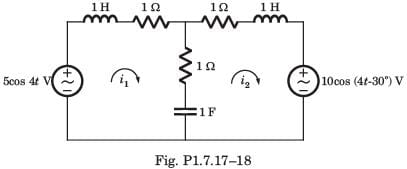Q.i2(t) = ?

Solution: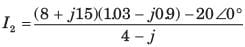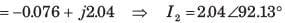QUESTION: 2

### I x = ?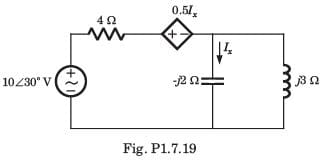Solution: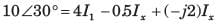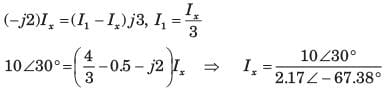QUESTION: 3

### Vx = ?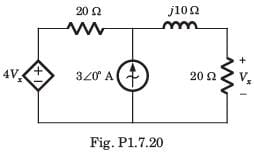Solution:

Let Vo be the voltage across current source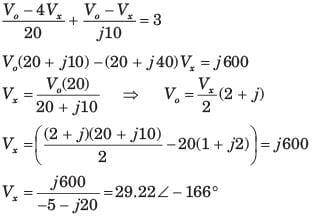QUESTION: 4

Determine the complex power for the given values in question.
Q. P = 269 W, Q = 150 VAR (capacitive)

Solution:

S = P - jQ = 269 - j150 VA

QUESTION: 5

Determine the complex power for the given values in question.
Q. Q = 2000 VAR, pf = 0.9 (leading)

Solution: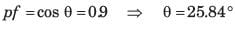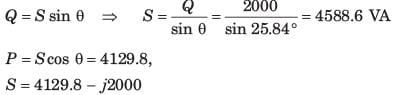QUESTION: 6

Determine the complex power for the given values in question.
Q. S = 60 VA, Q = 45 VAR (inductive)

Solution: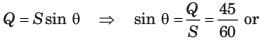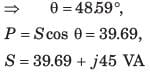QUESTION: 7

Determine the complex power for the given values in question.
Q. Vrms = 220 V, P = 1 kW, |Z| = 40 Ω (inductive)

Solution: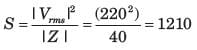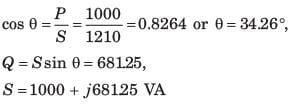QUESTION: 8

Determine the complex power for the given values in question.
Q. Vrms = 21∠ 200V, Vrms = 21∠200V, I rms = 8.5∠-500A

Solution: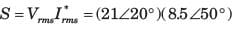= 61+ j167.7VA

QUESTION: 9

Determine the complex power for the given values in question.
Q. Vrms = 120∠ 300V, Z = 40 + j 80Ω

Solution: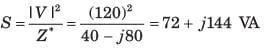QUESTION: 10

Vo = ?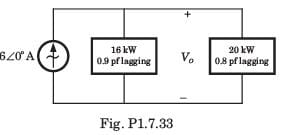Solution: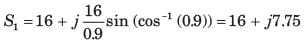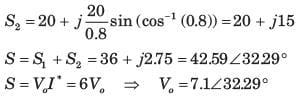Use Code STAYHOME200 and get INR 200 additional OFF Use Coupon Code